# KSEEB SSLC Class 10 Maths Solutions Chapter 12 Some Applications of Trigonometry Ex 12.1

KSEEB SSLC Class 10 Maths Solutions Chapter 12 Some Applications of Trigonometry Ex 12.1 are part of KSEEB SSLC Class 10 Maths Solutions. Here we have given Karnataka SSLC Class 10 Maths Solutions Chapter 12 Some Applications of Trigonometry Exercise 12.1.

## Karnataka SSLC Class 10 Maths Solutions Chapter 12 Some Applications of Trigonometry Exercise 12.1

Question 1.
A circus artist is climbing a 20 m long rope, which is tightly stretched and tied from the top of a vertical pole to the ground. Find the height of the pole, if the angle made by the rope with the ground level is 30°. (see the fig. given)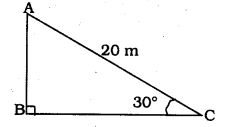Solution:
Length of rope is 20 m.
Height of the pole = ?, AB = ?
Let the height of the pole AB be ‘A’.
Length of rope AC = l = 20 m.
Angle of elevation, ∠BCA = 30°.
∴ Let ⊥∆AABC, ∠B = 90°.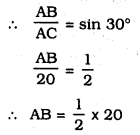∴ AB = 10 m.
∴ Height of the pole 10 m.

Question 2.
A tree breaks due to storm and the broken part bends, so that the tope of the tree touches the ground making an angle 30° with it. The distance between the foot of the tree to the point where the top touches the ground is 8 m. Find the height of the tree.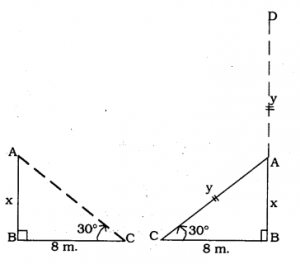Solution:
Let the Height of the broken part be ‘x’ m.
BC is the distance between the foot of the tree to the point where the top touches the ground.
In ⊥∆ABC, ∠B = 90°.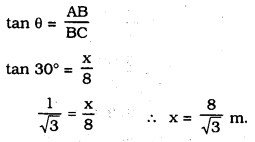∴ Height of broken part of the tree, AB = x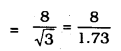= 4.62 m
Let AC = AD = y m.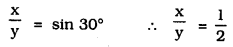∴ y = 2x = 2 × 4.62
y = 9.24 m.
Full height of the tree = x + y
∴ Height of the tree = x + y
= 4.62 + 9.24
= 13.86 m.

Question 3.
A contractor plans to install two slides for the children to play in a park. For the children below the age of 5 years, she prefers to have a slide whose top is at a height of 1.5 m, and is inclined at an angle of 30° to the ground, whereas for elder children, she wants to have a steep slide at a height of 3 m, and inclined at an angle of 60° to the ground. What should be the length of the slide in each case ?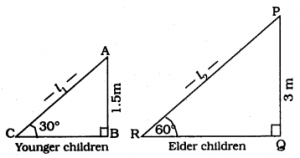Solution:
i) AB = 1.5 m., AC = l1 m., ∠ACB = 30°
In ⊥∆ABC, ∠B = 90°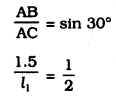∴ l1 = 2 × 1.5 = 3 m
∴ Length of slide of younger children = 3 m
ii) PQ = 3 m, OP = 12 m, ∠POQ = 60°
In ⊥∆PQR, ∠Q = 90°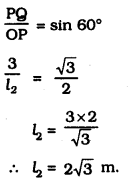∴ Length of slide of elder children = $$2 \sqrt{3}$$ m

Question 4.
The angle of elevation of the top of a tower from a point on the ground, which is 30 m away from the foot of the tower, is 30°. Find the height of the tower.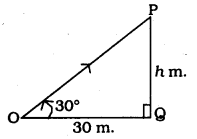Solution:
Let the height of a tower. PQ = h m.
Distance of QO from base of tower = 30 m.
Angle of elevation, ∠POQ = 30°
In ⊥∆APQO, ∠Q = 90°,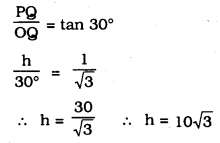∴ Height of tower, PQ = h = $$10 \sqrt{3}$$ m.

Question 5.
A kite is flying at a height of 60m above the ground. The string attached to the kite is temporarily tied to a point on the ground. The inclination of the string with the ground is 60°. Find the length of the string, assuming that there is no slack in the string.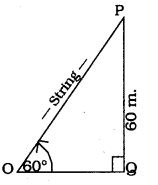Solution:
P is the position of Kite. It is flying at a height of 60 m. above the ground.
The inclination of the string with the ground, ∠POQ = 60°
Let length of string be OP = L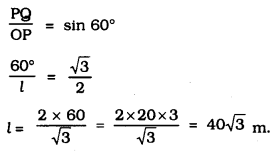∴ Length of slide of elder children $$40 \sqrt{3}$$ m.

Question 6.
A 1.5 m. tall boy is standing at some distance from a 30 m. tall building. The angle of elevation from his eyes to the top of the building increases from 30° to 60° as he walks towards the building. Find the distance he walked towards the building.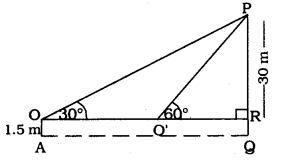Solution:
Height of the building, PQ = 30 m.
Height of the boy, OA = 1.5 m.
If the position of Boy at OA, angle of elevation, ∠POR = 30°, His second position, OO’, ∠POR = 60°.
RQ = OA = 1.5 m.
∴ PR = 30 – RQ = 30 – 1.5
PR = 28.5 m.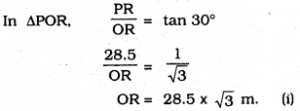Distance he walked towards the building:
OO’ = OR – O’R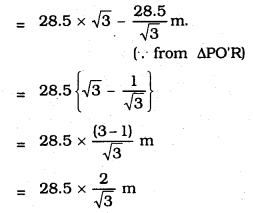Distance he walked towards the building:
OO’ = $$19 \sqrt{3}$$ m

Question 7.
From a point on the ground, the angles of elevation of the bottom and the top of a transmission tower fixed at the top of a 20 m high building are 45° and 60° respectively. Find the height of the tower.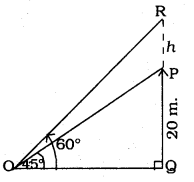Solution:
Let the height of building, PQ = 20 m.
PR= h m.
Bottom of transmission tower, P, vertex point ‘R’.
∠POQ = 45°, ∠ROQ = 60°.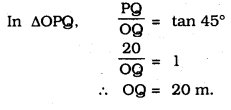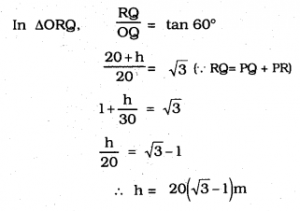∴ Height of transmission tower is = $$20(\sqrt{3}-1)$$ m

Question 8.
A statue, 1.6 m tall, stands on the top of a pedestal. From a point on the ground, the angle of elevation of the top of the statue is 60° and from the same point the angle of elevation of the top of the pedestal is 45°. Find the height of the pedestal.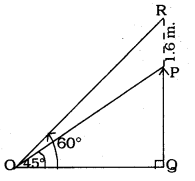Solution:
Height of pedestal, PQ = h m.
PR = 1.6 m.
∠POQ = 45°
∠ROQ = 60°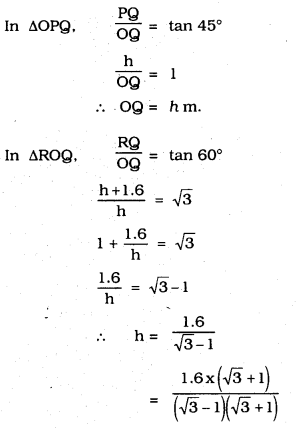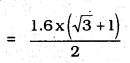∴ Height of pedestal, h = $$0.8 \times(\sqrt{3}+1)$$ m

Question 9.
The angle of elevation of the top of a building from the foot of the tower is 30° and the angle of elevation of the top of the tower from the foot of the building is 60°. If the tower is 50 m high. find the height of the building.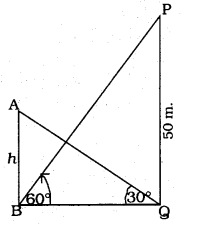Solution:
Height of the tower, PQ = 50 m.
Let Height of the building, AB = h m.
Angle of elevation to top of tower, = ∠PBQ = 60°
Angle of elevation to building. = ∠AQB = 30°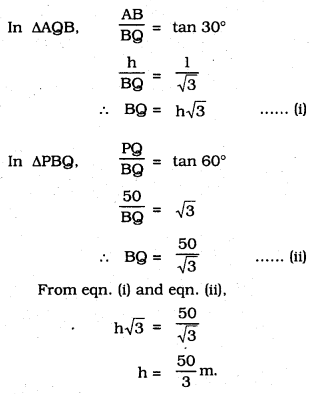h = 16.6 m

Question 10.
Two poles of equal heights are standing opposite each other on either side of the road, which is 80 m wide. From a point between them on the road, the angles of elevation of the top of the poles are 60° and 30°, respectively. Find the height of the poles and the distances of the point from the poles.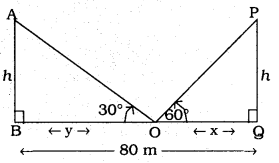Solution:
Height of AB and PQ be h m.
‘O’ is the point on road.
∠POQ = 60°, ∠AOB = 30°
OQ = ‘x’ m. OB = ‘y’ m.
x + y = 80 m.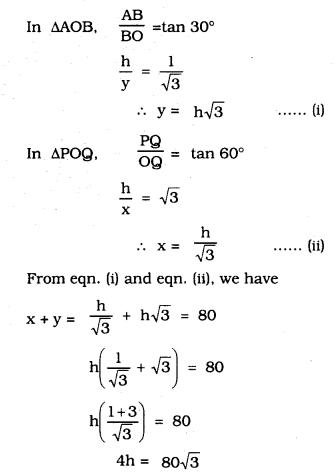∴ Height of pole, AB = PQ = h = $$20 \sqrt{3}$$ m.
Distance of the points c from the poles 20 m. and 60 m.

Question 11.
A TV tower stands vertically on a bank Of a canal. From a point on the other bank directly opposite the tower, the angle of elevation of the top of the tower is 60°. From another point 20 away from this point on the line joining this point to the foot of the tower, the angle of elevation of the top of the tower is 30° (see the figure given). Find the height of the tower and the width of the canal.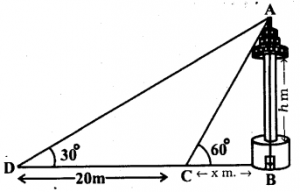Solution:
Let the height of TV tower, AB = h m.
Breadth of a canal, BC = x m.
∠ACB = 60° ∠ADB = 30°,
DC = 20 m..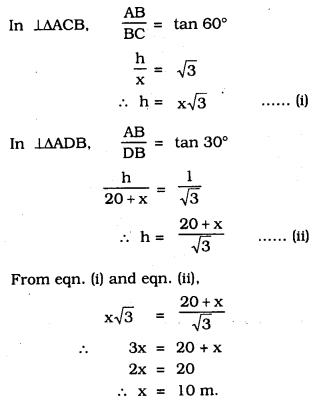From eqtn. (ii).
$$\mathrm{h}=\mathrm{x} \sqrt{3}=10 \sqrt{3}$$
∴ Height of tower = $$10 \sqrt{3}$$ m
Breadth of canal = 10 m.

Question 12.
From the top of a 7m. high building, the angle of elevation of the top of a cable tower is 60° and th angle of depression is its foot is 45°. Determine the height of the tower.
Solution:
Let the height of tower, PQ = h m.
The height of building, AB = 7 m.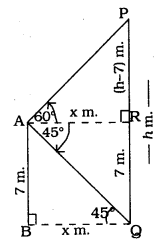Angle of elevation, ∠PAR = 60°
Angle of depression, ∠RAQ = 45°
∠AQB = 45° (∵ Alternate angle)
Let, BQ = AR = x m.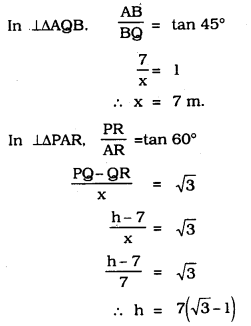∴ Height of tower, PQ = $$7(\sqrt{3}-1)$$ m.

Question 13.
As observed from the top of a 75 m. high lighthouse from the sea level, the angles of depression of two ships are 30° and 45°. If one ship is exactly behind the other on the same side of the lighthouse, find the distance between the two ships.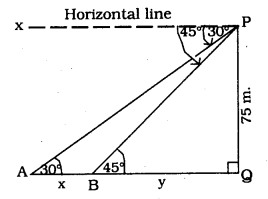Solution:
Height of lighthouse, PQ = 75 m.
A and B are positions of ship.
Px is Horizontal line.
∠APx = 30° and ∠BPx = 45°
∠PAQ = 30° and ∠PBQ = 45°
Let AB = x m, BQ = y m.
Then AQ = AB + BQ = (x + y) m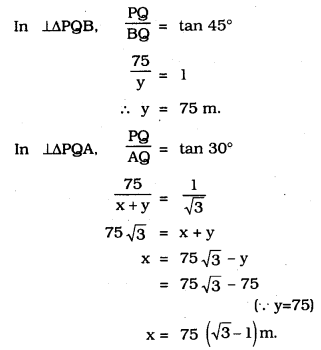∴ Distance between the two ships, AQ = x + y
= $$75 \sqrt{3}$$ m.

Question 14.
A 1.2 m tall girl spots a balloon moving with the wind in a horizontal line at a height of 88.2 m from the ground. The angle of elevation of the balloon from the eyes of the girl at any instant is 60°. After some time, the angle of elevation reduces to 30° (see the figure given below). Find the distance travelled by the balloon during the interval.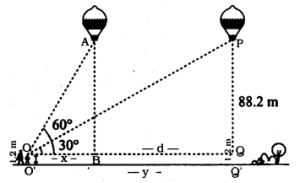Solution:
Height of the girl, OO’ = 1.2 m
∠AOB = 60°
∠POQ = 30°
Let OB = x m, BQ = d m, O’Q’ = y m.
AB = PQ = Q’P – Q’Q
= 88.2 – O’O
= 88.2 – 1.2 = 87 m.
Let OQ = ‘y’.
Distance balloon travelled, d = BQ
= (y – x)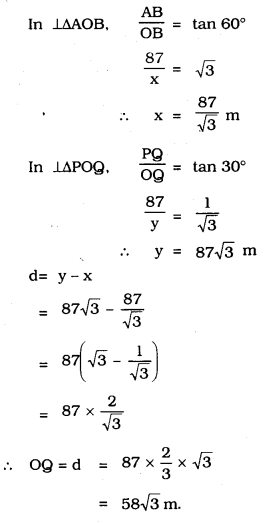Question 15.
A straight highway leads to the foot of a tower. A man standing at the top of the tower observes a car at an angle of depression of 30°, which is approaching the foot of the tower with a uniform speed. Six seconds later, the angle of depression of the car is found to be 60°. Find the time taken by the car to reach the foot of the tower from this point.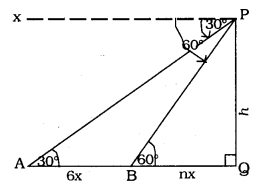Solution:
Let the height of tower, PQ = h m.
Px = Horizontal line
Let the positions of 1st car and 2nd car be A and B.
∠APx = 30° ∴ ∠PAQ = 30°
∠BPx = 60° ∠PBQ = 60°
Let speed of car be x m/s.
∴ Distance, AB = 6x m.
Time required to travel from B to Q be ‘n’ seconds,
∴ Distance, BQ = nx m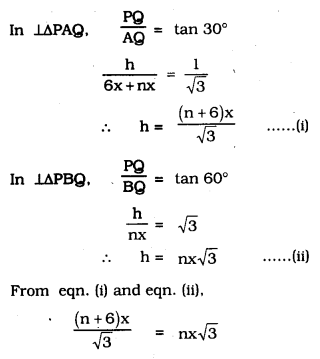$$n+6=n \sqrt{3} \times \sqrt{3}$$
3n = n+6
2n=6
∴ n = 3 seconds

Question 16.
The angles of elevation of the top of a tower from two points at a distance of 4m and 9m from the base of the tower and in the same straight line with it are complementary. Prove that the height of the tower is 6m.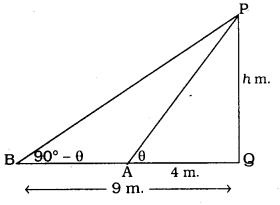Solution:
Let the height of tower, PQ = h m.
AQ = 4 m, BQ = 9 m.
∠PAQ = θ ∠PBQ = 90 – θ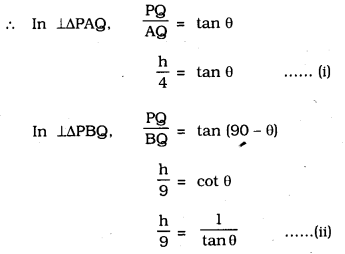From eqn. (i) and eqn. (ii),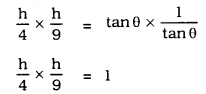∴ h2 = 36
∴ h = 6 m
∴ Height of tower, h = 6 m.

We hope the given KSEEB SSLC Class 10 Maths Solutions Chapter 12 Some Applications of Trigonometry Ex 12.1 will help you. If you have any query regarding Karnataka SSLC Class 10 Maths Solutions Chapter 12 Some Applications of Trigonometry Exercise 12.1, drop a comment below and we will get back to you at the earliest.